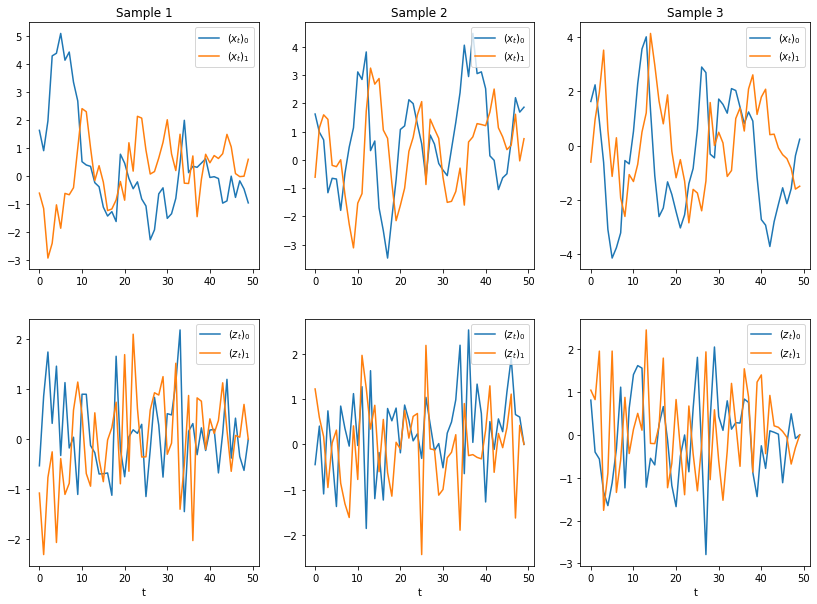# Basics: Noise

Here we continue our introduction to linear dynamical systems. In Part 1, we discussed state and dynamics. In Part 2, we will discuss noise.

Recall that a linear dynamical system is described by a time dependent state $$x_t \in \mathcal{R}^{n}$$ and a dynamics matrix $$A \in \mathcal{A}^{n \times n}$$, which does not depend on time. The system updates according to the equation $$x_{t+1} = A x_t$$. Such a system is fully deterministic and time reversible. Meaning, given the state $$x_t$$ at time $$t$$, the state at time $$t-1$$ is given by $$A^{-1}x_t$$ (assuming $$A$$ is invertible).

Realistic linear dynamical systems include a non-deterministic component, which we call noise. Noise enters the dynamics at time $$t$$ as a vector $$z_t \in \mathcal{R}^n$$ added to the state update

$x_{t+1} = Ax_t + z_t.$

The noise $$z_t$$ is drawn from some probability distribution, usually $$\mathcal{N}(0,\sigma^2I)$$. In other words, each entry of $$z_t$$ is a mean zero Gaussian random variable with variance $$\sigma^2$$, and the entries are uncorrelated.

With the addition of noise, the system is no longer reversible. There are many combinations of $$x_{t-1}$$ and $$z_{t-1}$$ that could have produced a given $$x_t$$. Of course the future state is no longer certain either.

To illustrate the effect of noise, we show several sample trajectories of the same linear dynamical system with different realizations of the noise vectors $$z_1,\ldots,z_T$$.

## Code and plots

import numpy as np
import matplotlib.pyplot as plt
%matplotlib inline

# Show sample trajectories under different noise realizations.
T = 50
n = 2
np.random.seed(1)
A = np.array([[.74, -.37], [.21, .70]])
x = np.zeros((n, T))
x[:, 0] = np.random.randn(n)

sigma = 1.0
nsamples = 3
plt.figure(figsize=(12, 8))
for j in range(nsamples):
z = np.zeros((n, T))
for t in range(T-1):
z[:, t] = np.random.normal(0.0, sigma, n)
x[:, t+1] = A @ x[:, t] + z[:, t]
plt.subplot(2, nsamples, j+1)
plt.plot(x[0, :], label=r"$(x_t)_0$")
plt.plot(x[1, :], label=r"$(x_t)_1$")
plt.title("Sample %i" % (j+1))
plt.legend(loc="upper right")

plt.subplot(2, nsamples, j+1+nsamples)
plt.plot(z[0, :], label=r"$(z_t)_0$")
plt.plot(z[1, :], label=r"$(z_t)_1$")
plt.xlabel("t")
plt.legend(loc="upper right")
plt.show()Written on October 2, 2020